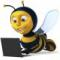# Swap two variables

2 posts / 0 new
#1 9 January, 2011 - 20:24
Karthik#### Swap two variables

stuartjems Wrote: Hey all
I have one problem and i am trying to find solution of that .
"swap two variables without using third temporary variable"
If anyone know then please let me know!

Following methods available:
I had tested some of the methods below in C, C++.
But, nothing works in vb.
1)
a^=b^=a^=b

2)
a = a # b
b = a @ b
a = a @ b
(Not works in C)
3)
In Ruby
a,b = b,a
4)
In PHP
list(\$a, \$b) = array(\$b, \$a);

5) javascript
[a, b] = [b, a]

6)
a = a + b
b = a - b
a = a - b

7)
In C / C++
a += b;
b = a - b;
a = a - b;

8)
a = a + b - (b = a);

source: stackoverflow.com

If you find anything works in VB, post below.

28 November, 2011 - 16:37
Charles ElenaI think you can try this one. You can do using Xor function.a = a Xor bb = a Xor ba = a Xor b Do write function and rest of the things.